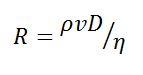# Reynolds Number Calculator

This CalcTown calculator calculates Reynold's number to predict whether the flow of a fluid will be laminar or turbulent.

m
Kg/m3
N.s/m2
m/s

#### Resultwhere

NR: Reynolds Number, dimensionless
ρ: Fluid Density, in Kg/m^3
v: Fluid Speed (velocity), in meter/second
D: Pipe Diameter (travelled length of the fluid; hydraulic diameter in river system), in meter
η: Fluid Viscosity, in N.s/m^2